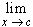#Interactive Real Analysis

Next | Previous | Glossary | Map

## 6.5. Differentiable Functions

### Theorem 6.5.5: Differentiable and Continuity

If f is differentiable at a point c, then f is continuous at that point c. The converse is not true.

### Proof:

Note that

• f(x) - f(c) =( x - c )

As x approaches c, the limit of the quotient exists by assumption and is equal to f'(c), and the limit of the right-hand factor exists also and is zero. Therefore:

•f(x) - f(c) = 0

which is another way of stating that f is continuous at x = c.

Next | Previous | Glossary | Map